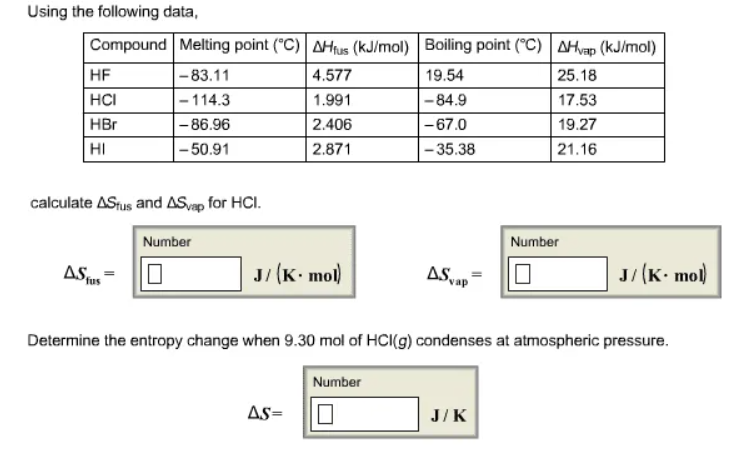# Problem: Using the following data, calculate ΔSfus and ΔSvap for HCl. Determine the entropy change when 9.30 mol of HCl(g) condenses at atmospheric pressure.

###### FREE Expert Solution

Calculate ΔSfus:

100% (327 ratings)###### Problem Details

Using the following data, calculate ΔSfus and ΔSvap for HCl. Determine the entropy change when 9.30 mol of HCl(g) condenses at atmospheric pressure.